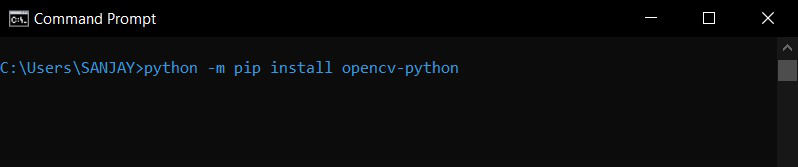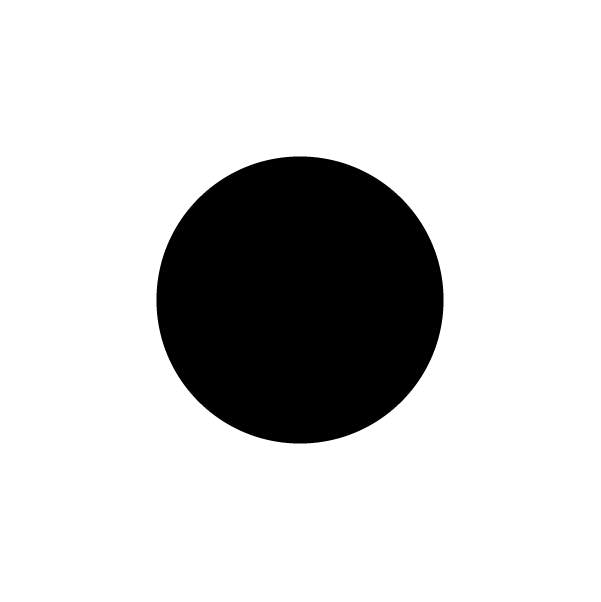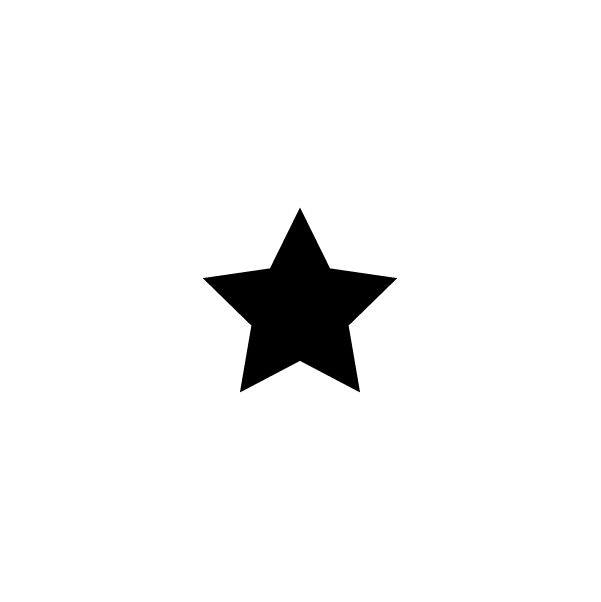Open in App
Not now

# How to subtract two images using Python-OpenCV ?

• Last Updated : 03 Jan, 2023

In this article, we are going to see how to subtract two images using OpenCV in Python.

Now, before getting into the topic we shall discuss some of the use cases of Arithmetic operations. Arithmetic operations like addition and subtraction can help us to make images brighter or darker. Specifically, subtraction of two images has a lot of purposes if you are up to find the difference between the two similar-looking images or comparing this comes in handy. OpenCV checks or manipulates the images by pixel level because of this fact we can get the difference of the images in pixel level.

## Syntax:

`Syntax:  cv2.subtract(image1, image2)`

Note: Before you subtract any image, you should note that the two images must be in the same size and depth. Otherwise, it will throw an error.

## Installation

To install OpenCV, type the below command in the terminal.

```python3 -m pip install opencv--python
or
pip install opencv-python```By installing OpenCV it will automatically install NumPy on your system. So you are good to go. Now let’s see how to subtract two images using OpenCV and python.

## Python3

 `# importing opencv``import` `cv2`

### Step 2: Read the images

Next, we need to read the images first to use the images in the program.

## Python3

 `# reading the images``circle ``=` `cv2.imread(``'circle.png'``)``star ``=` `cv2.imread(``'star.png'``)`

### Step 3: Subtract the images

Now, we can subtract the images by the inbuilt cv2 method called cv2.subtract

Syntax:  cv2.subtract(image1, image2)

## Python3

 `# subtract the images``subtracted ``=` `cv2.subtract(star, circle)`

### Step 4: Show the output

For showing the images we need to do 3 things first showing the image by cv2.imshow()

Syntax: cv2.imshow(“name of the window”, image)

The next two lines of code assure us to give us an option to close the shown image.

cv2.waitKey(0) -> will wait for the infinite time for you to press any key in the keyboard

cv2.destroyAllWindows() -> will close all the windows

## Python3

 `# TO show the output``cv2.imshow(``'image'``, subtracted)`` ` `# To close the window``cv2.waitKey(``0``)``cv2.destroyAllWindows()`

Here is the python code to subtract two images in python,

Input Images:## Python3

 `# importing opencv``import` `cv2`` ` `# reading the images``circle ``=` `cv2.imread(``'circle.png'``)``star ``=` `cv2.imread(``'star.png'``)`` ` `# subtract the images``subtracted ``=` `cv2.subtract(star, circle)`` ` `# TO show the output``cv2.imshow(``'image'``, subtracted)`` ` `# To close the window``cv2.waitKey(``0``)``cv2.destroyAllWindows()`

Output:## Applications

• To convert image into PNG – Image subtraction using OpenCV is used to remove background images and convert them into png.
• To Know differences between two images – If we have two similar images with some differences. And we want to know the differences, we can go for this image subtraction to find it out.
• Image Brightness and contrast – Adding or subtracting some pixels will adjust the brightness and contrast of the image.
• To adjust illumination gradient in the image – If an image containing text is been badly illuminated. It is hard for us to read it. Image Subtraction makes it easy to read the text in that paper.
• Instagram and Snapchat filters – Yes, The filters which we use to take different selfies and photos use image subtraction.

My Personal Notes arrow_drop_up